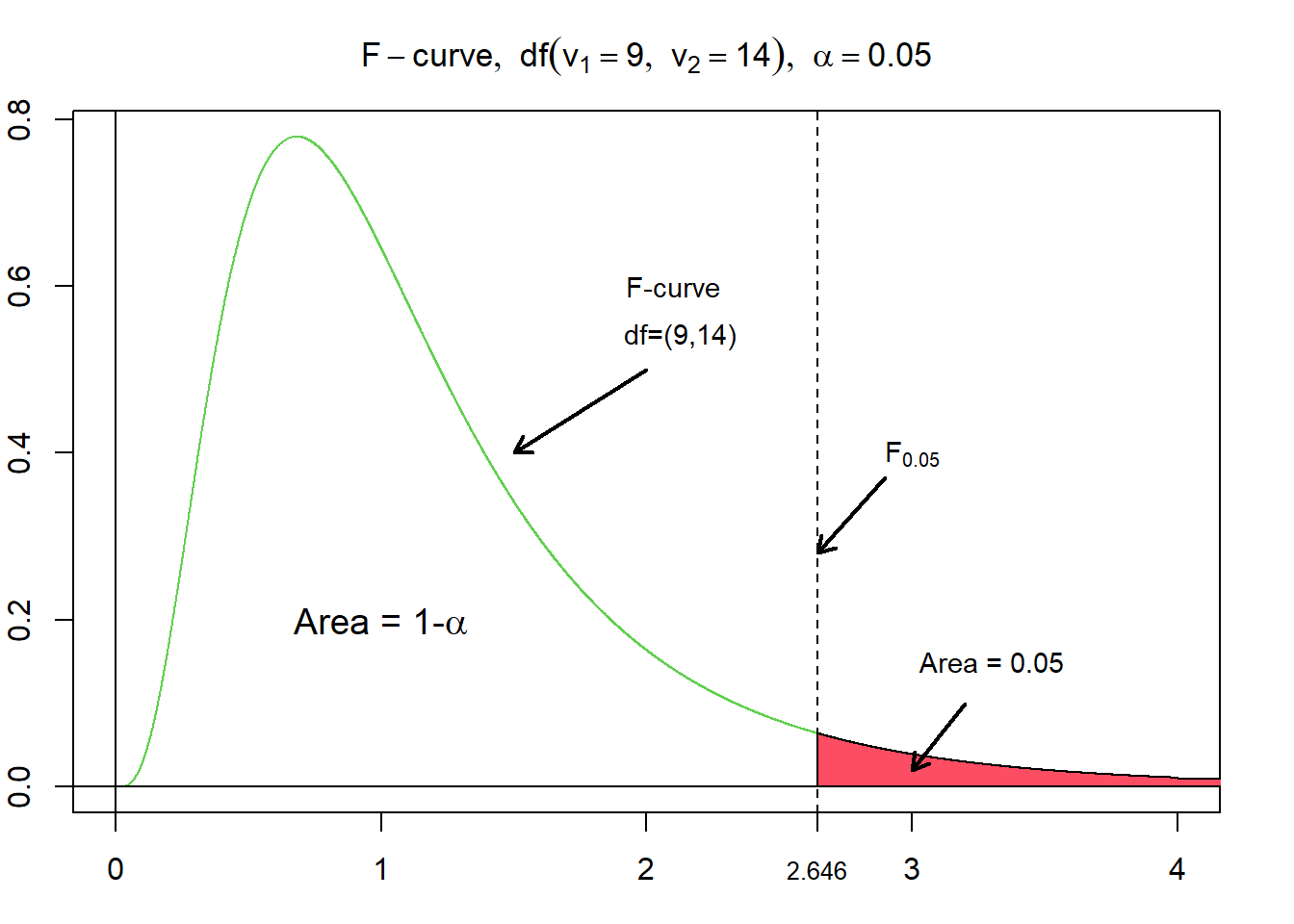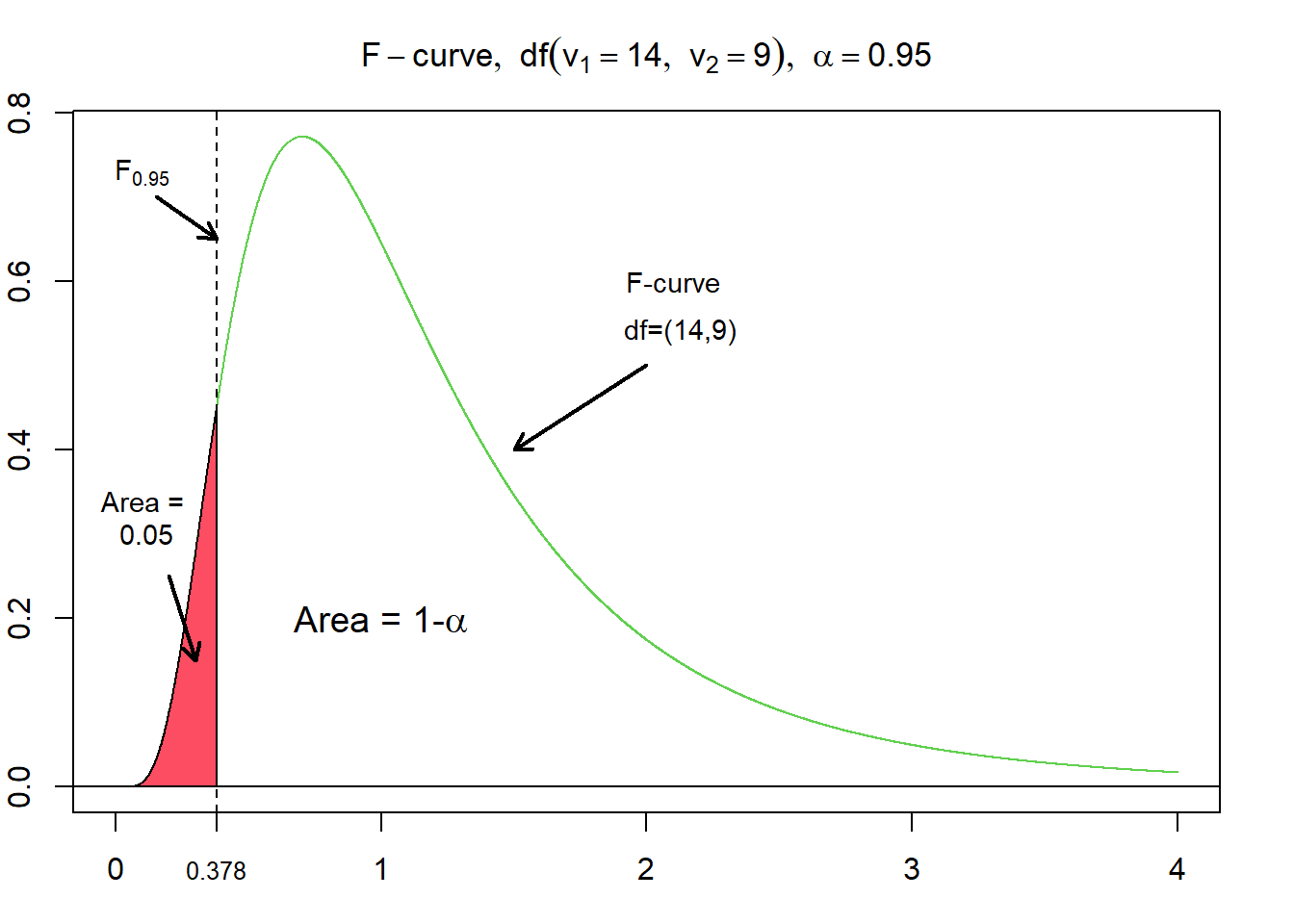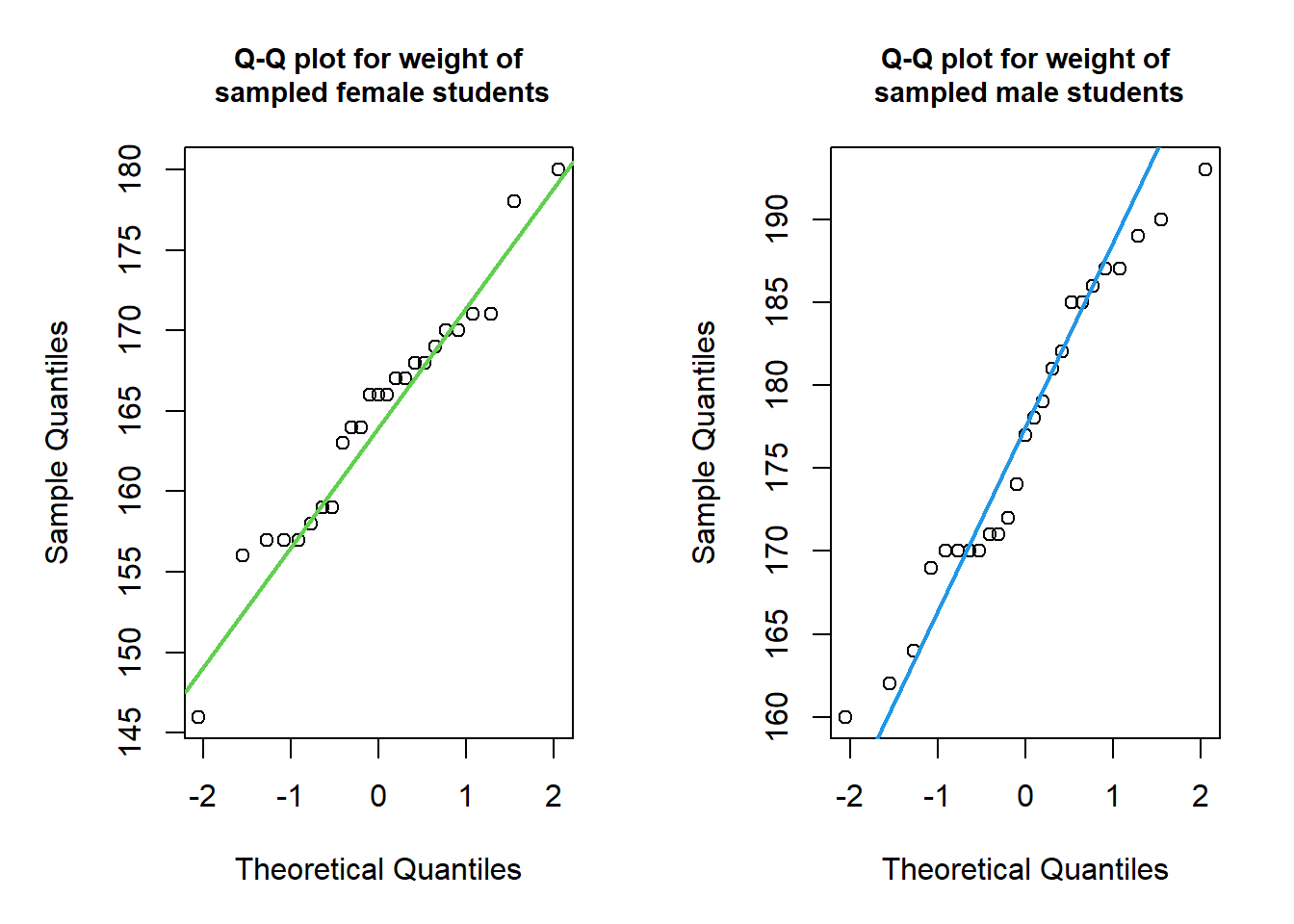In this section we discuss hypothesis tests for two population standard deviations. In other words, we discuss methods of inference for the standard deviations of one variable from two different populations. These methods are based on the $$F$$-distribution, named in honor of Sir Ronald Aylmer Fisher.

The $$F$$-distribution is a right-skewed probability density distribution with two shape parameters, $$v_1$$ and $$v_2$$, called the degrees of freedom for the numerator ($$v_1$$) and the degrees of freedom for the denominator ($$v_2$$).

$df = (v_1,v_2)$

As for any other density curve the area under the curve of the $$F$$-distribution corresponds to probabilities. The area under the curve and thus, the probability for any given interval and given $$df$$ is computed with software. Alternatively, one may look them up in a table. In those tables generally the degrees of freedom for the numerator ($$v_1$$) are displayed along the top, whereas the degrees of freedom for the denominator ($$v_2$$) are displayed in the outside column on the left.

In order to perform a hypothesis test for two population standard deviations, the value that corresponds to a specified area under a $$F$$-curve is calculated.

Given $$\alpha$$, where $$\alpha$$ corresponds to a probability between 0 and 1, $$F_{\alpha}$$ denotes the value having an area $$\alpha$$ to its right under a $$F$$-curve.In the figure above $$F_{0.05}$$ for $$df=(9,14)$$ evaluates to $$\approx 2.6458$$.

One interesting property of $$F$$-curves is the reciprocal property. This means, that for a $$F$$-curve with $$df = (v_1, v_2)$$, the $$F$$-value having the area $$\alpha$$ to its left equals the reciprocal of the $$F$$-value having the area $$\alpha$$ to its right for an $$F$$-curve with $$df = (v_2, v_1)$$ (Weiss, 2010). Adopted to the example from above, where $$F_{0.05}$$ for $$df=(9,14)$$ evaluates to $$\approx 2.6458$$, this means that $$F_{0.95}$$ for $$df=(14,9)$$ evaluates to $$\frac{1}{2.6458}=0.378$$.#### Interval Estimation of $$\sigma_1 - \sigma_2$$

The $$100(1-\alpha)$$ % confidence interval for $$\sigma$$ is

$\frac{1}{\sqrt{F_{\alpha /2}}} \times \frac{s_1}{s_2} \le \sigma \le \frac{1}{\sqrt{F_{1-\alpha /2}}} \times \frac{s_1}{s_2}\text{,}$

where $$s_1$$ and $$s_2$$ are the sample standard deviations.

#### Two Standard Deviations $$F$$-test

The hypothesis-testing procedure for two standard deviations is called two standard deviations $$F$$-test. Hypothesis testing for two population standard deviations follows the same step-wise procedure as for other hypothesis tests:

$\begin{array}{l} \hline \ \text{Step 1} & \text{State the null hypothesis } H_0 \text{ and alternative hypothesis } H_A \text{.}\\ \ \text{Step 2} & \text{Decide on the significance level, } \alpha\text{.} \\ \ \text{Step 3} & \text{Compute the value of the test statistic.} \\ \ \text{Step 4} &\text{Determine the p-value.} \\ \ \text{Step 5} & \text{If } p \le \alpha \text{, reject }H_0 \text{; otherwise, do not reject } H_0 \text{.} \\ \ \text{Step 6} &\text{Interpret the result of the hypothesis test.} \\ \hline \end{array}$

The test statistic for a hypothesis test for a normally distributed variable and for independent samples of sizes $$n_1$$ and $$n_2$$ is given by

$F = \frac{s_1^2/\sigma_1^2}{s_2^2/\sigma_2^2}\text{,}$ with $$df = (n_1 - 1,\; n_2 - 1)$$.

If $$H_0: \sigma_1 = \sigma_2$$ is true, then the equation simplifies to

$F = \frac{s_1^2}{s_2^2}\text{.}$

#### Two Standard Deviations $$F$$-test: An example

In order to get some hands-on experience we apply the two standard deviations $$F$$-test in an exercise. For this we load the students data set, which you may also download here.

students <- read.csv("https://userpage.fu-berlin.de/soga/data/raw-data/students.csv")

The students data set consists of 8239 rows, each of them representing a particular student, and 16 columns, each of them corresponding to a variable/feature related to that particular student. These self-explaining variables are: stud.id, name, gender, age, height, weight, religion, nc.score, semester, major, minor, score1, score2, online.tutorial, graduated, salary.

In order to showcase the two standard deviations $$F$$-test we examine once again the height variable in the students data set. We want to test, if the standard deviation of the height of female students ($$\sigma_1$$) is different from the standard deviation of the height of male students ($$\sigma_2$$).

#### Data preparation

• We subset the data set based on the variable gender.
• We sample 25 female students and 25 male students.
• We calculate the standard deviations of the variable of interest (height in cm) for both samples and assign them the variables sd_female and sd_male.
female <- subset(students, gender == "Female")
male <- subset(students, gender == "Male")

n <- 25
female_sample <- sample(female$height, n) male_sample <- sample(male$height, n)

sd_female <- sd(female_sample)
sd_female
##  7.414625
sd_male <- sd(male_sample)
sd_male
##  9.293008

Further, we check the normality assumption by plotting a Q-Q plot. In R we apply the qqnorm() and the qqline() functions for plotting Q-Q plots.

par(mfrow = c(1, 2), mar = c(5, 5, 4, 2))

# sample data females
qqnorm(female_sample, main = "Q-Q plot for weight of\n sampled female students", cex.main = 0.9)
qqline(female_sample, col = 3, lwd = 2)

# sample data males
qqnorm(male_sample, main = "Q-Q plot for weight of\n sampled male students", cex.main = 0.9)
qqline(male_sample, col = 4, lwd = 2)The data of both samples falls roughly onto a straight line. Based on the graphical evaluation approach we conclude that the data is normally distributed.

#### Hypothesis testing

In order to conduct the two standard deviations $$F$$-test we follow the step-wise implementation procedure for hypothesis testing.

Step 1: State the null hypothesis $$H_0$$ and alternative hypothesis $$H_A$$

The null hypothesis states that the standard deviation of the height of female students ($$\sigma_1$$) equals the standard deviation of the height of male students($$\sigma_2$$):

$H_0: \quad \sigma_1 = \sigma_2$

Alternative hypothesis:

$H_A: \quad \sigma_1 \ne \sigma_2$

This formulation results in a two-sided hypothesis test.

Step 2: Decide on the significance level, $$\alpha$$

$\alpha = 0.05$

alpha <- 0.05

Step 3 and 4: Compute the value of the test statistic and the p-value

For illustration purposes we manually compute the test statistic in R. Recall the equation for the test statistic from above:

$F = \frac{s_1^2}{s_2^2}$

# compute the value of the test statistic
Ftest <- sd_female^2 / sd_male^2
Ftest
##  0.6365987

The numerical value of the test statistic is $$\approx 0.64$$.

In order to calculate the p-value we apply the pf() function. Recall how to calculate the degrees of freedom:

$df = (n_1 - 1, n_2 - 1)$

# compute df
df1 <- length(female_sample) - 1
df2 <- length(male_sample) - 1

# compute the p-value
p_upper <- pf(Ftest, df1 = df1, df2 = df2, lower.tail = FALSE)
p_lower <- pf(Ftest, df1 = df1, df2 = df2, lower.tail = TRUE)

if (p_upper * 2 > 1) {
p <- p_lower * 2
} else {
p <- p_upper * 2
}
p
##  0.2756173

$$p = 0.2756173$$.

Step 5: If $$p \le \alpha$$, reject $$H_0$$; otherwise, do not reject $$H_0$$

p <= alpha
##  FALSE

The p-value is greater than the specified significance level of 0.05; we do not reject $$H_0$$. The test results are statistically significant at the 5 % level and do not provide sufficient evidence against the null hypothesis.

Step 6: Interpret the result of the hypothesis test

At the 5 % significance level the data does not provide sufficient evidence to conclude, that the standard deviations of the height of female and male students is different.

#### Hypothesis testing in R

We just completed a two standard deviations $$F$$-test in R manually. OK, we learned a lot, but now we make use of the R machinery to obtain the same result as above using just one line of code!

In order to conduct a two standard deviations $$F$$-test in R we apply the var.test() function. We provide two vectors as data input female_sample and male_sample. We do not need to specify the alternative argument, as alternative = 'two.sided' is the default.

var.test(female_sample, male_sample)
##
##  F test to compare two variances
##
## data:  female_sample and male_sample
## F = 0.6366, num df = 24, denom df = 24, p-value = 0.2756
## alternative hypothesis: true ratio of variances is not equal to 1
## 95 percent confidence interval:
##  0.2805293 1.4446190
## sample estimates:
## ratio of variances
##          0.6365987

Worked out fine! Compare the output of the var.test() function to our result from above. Again, we may conclude that at the 5 % significance level the data does not provide sufficient evidence to conclude, that the standard deviations of the height of female and male students is different.

Citation

The E-Learning project SOGA-R was developed at the Department of Earth Sciences by Kai Hartmann, Joachim Krois and Annette Rudolph. You can reach us via mail by soga[at]zedat.fu-berlin.de.You may use this project freely under the Creative Commons Attribution-ShareAlike 4.0 International License.

Please cite as follow: Hartmann, K., Krois, J., Rudolph, A. (2023): Statistics and Geodata Analysis using R (SOGA-R). Department of Earth Sciences, Freie Universitaet Berlin.